# bezier.hazmat.geometric_intersection module¶

Helpers for intersecting Bézier curves via geometric methods.

The functions here are pure Python and many have equivalent implementations written in Fortran and exposed via a Cython wrapper.

bezier.hazmat.geometric_intersection.bbox_intersect(nodes1, nodes2)

Bounding box intersection predicate.

Note

There is also a Fortran implementation of this function, which will be used if it can be built.

Determines if the bounding box of two sets of control points intersects in $$\mathbf{R}^2$$ with non-trivial intersection (i.e. tangent bounding boxes are insufficient).

Note

Though we assume (and the code relies on this fact) that the nodes are two-dimensional, we don’t check it.

Parameters
• nodes1 (numpy.ndarray) – Set of control points for a Bézier shape.

• nodes2 (numpy.ndarray) – Set of control points for a Bézier shape.

Returns

Enum from BoxIntersectionType indicating the type of bounding box intersection.

Return type

int

bezier.hazmat.geometric_intersection.linearization_error(nodes)

Compute the maximum error of a linear approximation.

Note

There is also a Fortran implementation of this function, which will be used if it can be built.

Note

This is a helper for Linearization, which is used during the curve-curve intersection process.

We use the line

$L(s) = v_0 (1 - s) + v_n s$

and compute a bound on the maximum error

$\max_{s \in \left[0, 1\right]} \|B(s) - L(s)\|_2.$

Rather than computing the actual maximum (a tight bound), we use an upper bound via the remainder from Lagrange interpolation in each component. This leaves us with $$\frac{s(s - 1)}{2!}$$ times the second derivative in each component.

The second derivative curve is degree $$d = n - 2$$ and is given by

$B''(s) = n(n - 1) \sum_{j = 0}^{d} \binom{d}{j} s^j (1 - s)^{d - j} \cdot \Delta^2 v_j$

Due to this form (and the convex combination property of Bézier Curves) we know each component of the second derivative will be bounded by the maximum of that component among the $$\Delta^2 v_j$$.

For example, the curve

$\begin{split}B(s) = \left[\begin{array}{c} 0 \\ 0 \end{array}\right] (1 - s)^2 + \left[\begin{array}{c} 3 \\ 1 \end{array}\right] 2s(1 - s) + \left[\begin{array}{c} 9 \\ -2 \end{array}\right] s^2\end{split}$

has $$B''(s) \equiv \left[\begin{array}{c} 6 \\ -8 \end{array}\right]$$ which has norm $$10$$ everywhere, hence the maximum error is

$\left.\frac{s(1 - s)}{2!} \cdot 10\right|_{s = \frac{1}{2}} = \frac{5}{4}.$>>> nodes = np.asfortranarray([
...     [0.0, 3.0, 9.0],
...     [0.0, 1.0, -2.0],
... ])
>>> linearization_error(nodes)
1.25


As a non-example, consider a “pathological” set of control points:

$\begin{split}B(s) = \left[\begin{array}{c} 0 \\ 0 \end{array}\right] (1 - s)^3 + \left[\begin{array}{c} 5 \\ 12 \end{array}\right] 3s(1 - s)^2 + \left[\begin{array}{c} 10 \\ 24 \end{array}\right] 3s^2(1 - s) + \left[\begin{array}{c} 30 \\ 72 \end{array}\right] s^3\end{split}$

By construction, this lies on the line $$y = \frac{12x}{5}$$, but the parametrization is cubic: $$12 \cdot x(s) = 5 \cdot y(s) = 180s(s^2 + 1)$$. Hence, the fact that the curve is a line is not accounted for and we take the worse case among the nodes in:

$\begin{split}B''(s) = 3 \cdot 2 \cdot \left( \left[\begin{array}{c} 0 \\ 0 \end{array}\right] (1 - s) + \left[\begin{array}{c} 15 \\ 36 \end{array}\right] s\right)\end{split}$

which gives a nonzero maximum error:

>>> nodes = np.asfortranarray([
...     [0.0, 5.0, 10.0, 30.0],
...     [0.0, 12.0, 24.0, 72.0],
... ])
>>> linearization_error(nodes)
29.25


Though it may seem that 0 is a more appropriate answer, consider the goal of this function. We seek to linearize curves and then intersect the linear approximations. Then the $$s$$-values from the line-line intersection is lifted back to the curves. Thus the error $$\|B(s) - L(s)\|_2$$ is more relevant than the underyling algebraic curve containing $$B(s)$$.

Note

It may be more appropriate to use a relative linearization error rather than the absolute error provided here. It’s unclear if the domain $$\left[0, 1\right]$$ means the error is already adequately scaled or if the error should be scaled by the arc length of the curve or the (easier-to-compute) length of the line.

Parameters

nodes (numpy.ndarray) – Nodes of a curve.

Returns

The maximum error between the curve and the linear approximation.

Return type

float

bezier.hazmat.geometric_intersection.segment_intersection(start0, end0, start1, end1)

Determine the intersection of two line segments.

Assumes each line is parametric

\begin{split}\begin{alignat*}{2} L_0(s) &= S_0 (1 - s) + E_0 s &&= S_0 + s \Delta_0 \\ L_1(t) &= S_1 (1 - t) + E_1 t &&= S_1 + t \Delta_1. \end{alignat*}\end{split}

To solve $$S_0 + s \Delta_0 = S_1 + t \Delta_1$$, we use the cross product:

$\left(S_0 + s \Delta_0\right) \times \Delta_1 = \left(S_1 + t \Delta_1\right) \times \Delta_1 \Longrightarrow s \left(\Delta_0 \times \Delta_1\right) = \left(S_1 - S_0\right) \times \Delta_1.$

Similarly

$\Delta_0 \times \left(S_0 + s \Delta_0\right) = \Delta_0 \times \left(S_1 + t \Delta_1\right) \Longrightarrow \left(S_1 - S_0\right) \times \Delta_0 = \Delta_0 \times \left(S_0 - S_1\right) = t \left(\Delta_0 \times \Delta_1\right).$

Note

Since our points are in $$\mathbf{R}^2$$, the “traditional” cross product in $$\mathbf{R}^3$$ will always point in the $$z$$ direction, so in the above we mean the $$z$$ component of the cross product, rather than the entire vector.

For example, the diagonal lines

\begin{split}\begin{align*} L_0(s) &= \left[\begin{array}{c} 0 \\ 0 \end{array}\right] (1 - s) + \left[\begin{array}{c} 2 \\ 2 \end{array}\right] s \\ L_1(t) &= \left[\begin{array}{c} -1 \\ 2 \end{array}\right] (1 - t) + \left[\begin{array}{c} 1 \\ 0 \end{array}\right] t \end{align*}\end{split}

intersect at $$L_0\left(\frac{1}{4}\right) = L_1\left(\frac{3}{4}\right) = \frac{1}{2} \left[\begin{array}{c} 1 \\ 1 \end{array}\right]$$.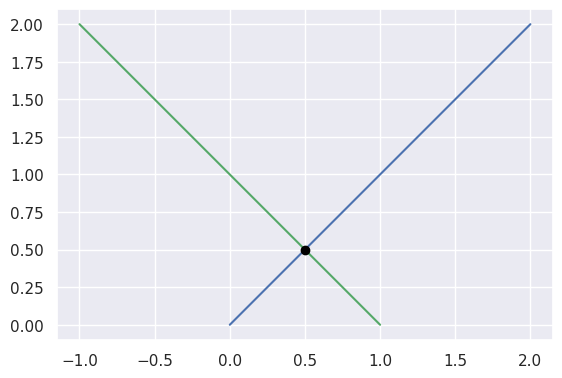>>> start0 = np.asfortranarray([0.0, 0.0])
>>> end0 = np.asfortranarray([2.0, 2.0])
>>> start1 = np.asfortranarray([-1.0, 2.0])
>>> end1 = np.asfortranarray([1.0, 0.0])
>>> s, t, _ = segment_intersection(start0, end0, start1, end1)
>>> s
0.25
>>> t
0.75


Taking the parallel (but different) lines

\begin{split}\begin{align*} L_0(s) &= \left[\begin{array}{c} 1 \\ 0 \end{array}\right] (1 - s) + \left[\begin{array}{c} 0 \\ 1 \end{array}\right] s \\ L_1(t) &= \left[\begin{array}{c} -1 \\ 3 \end{array}\right] (1 - t) + \left[\begin{array}{c} 3 \\ -1 \end{array}\right] t \end{align*}\end{split}

we should be able to determine that the lines don’t intersect, but this function is not meant for that check: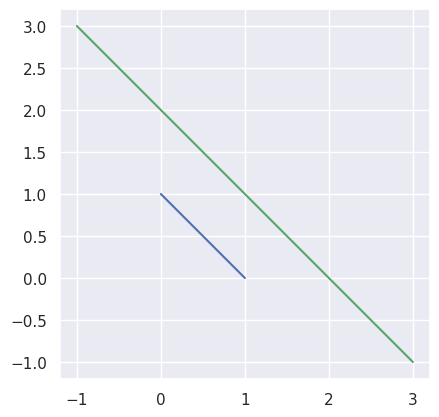>>> start0 = np.asfortranarray([1.0, 0.0])
>>> end0 = np.asfortranarray([0.0, 1.0])
>>> start1 = np.asfortranarray([-1.0, 3.0])
>>> end1 = np.asfortranarray([3.0, -1.0])
>>> _, _, success = segment_intersection(start0, end0, start1, end1)
>>> success
False


Instead, we use parallel_lines_parameters():

>>> disjoint, _ = parallel_lines_parameters(start0, end0, start1, end1)
>>> disjoint
True


Note

There is also a Fortran implementation of this function, which will be used if it can be built.

Parameters
• start0 (numpy.ndarray) – A 1D NumPy 2-array that is the start vector $$S_0$$ of the parametric line $$L_0(s)$$.

• end0 (numpy.ndarray) – A 1D NumPy 2-array that is the end vector $$E_0$$ of the parametric line $$L_0(s)$$.

• start1 (numpy.ndarray) – A 1D NumPy 2-array that is the start vector $$S_1$$ of the parametric line $$L_1(s)$$.

• end1 (numpy.ndarray) – A 1D NumPy 2-array that is the end vector $$E_1$$ of the parametric line $$L_1(s)$$.

Returns

Pair of $$s_{\ast}$$ and $$t_{\ast}$$ such that the lines intersect: $$L_0\left(s_{\ast}\right) = L_1\left(t_{\ast}\right)$$ and then a boolean indicating if an intersection was found (i.e. if the lines aren’t parallel).

Return type
bezier.hazmat.geometric_intersection.parallel_lines_parameters(start0, end0, start1, end1)

Checks if two parallel lines ever meet.

Meant as a back-up when segment_intersection() fails.

Note

This function assumes but never verifies that the lines are parallel.

In the case that the segments are parallel and lie on different lines, then there is a guarantee of no intersection. However, if they are on the exact same line, they may define a shared segment coincident to both lines.

In segment_intersection(), we utilized the normal form of the lines (via the cross product):

\begin{split}\begin{align*} L_0(s) \times \Delta_0 &\equiv S_0 \times \Delta_0 \\ L_1(t) \times \Delta_1 &\equiv S_1 \times \Delta_1 \end{align*}\end{split}

So, we can detect if $$S_1$$ is on the first line by checking if

$S_0 \times \Delta_0 \stackrel{?}{=} S_1 \times \Delta_0.$

If it is not on the first line, then we are done, the segments don’t meet: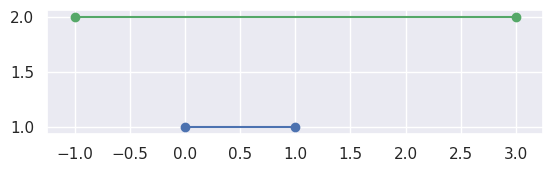>>> # Line: y = 1
>>> start0 = np.asfortranarray([0.0, 1.0])
>>> end0 = np.asfortranarray([1.0, 1.0])
>>> # Vertical shift up: y = 2
>>> start1 = np.asfortranarray([-1.0, 2.0])
>>> end1 = np.asfortranarray([3.0, 2.0])
>>> disjoint, _ = parallel_lines_parameters(start0, end0, start1, end1)
>>> disjoint
True


If $$S_1$$ is on the first line, we want to check that $$S_1$$ and $$E_1$$ define parameters outside of $$\left[0, 1\right]$$. To compute these parameters:

$L_1(t) = S_0 + s_{\ast} \Delta_0 \Longrightarrow s_{\ast} = \frac{\Delta_0^T \left( L_1(t) - S_0\right)}{\Delta_0^T \Delta_0}.$

For example, the intervals $$\left[0, 1\right]$$ and $$\left[\frac{3}{2}, 2\right]$$ (via $$S_1 = S_0 + \frac{3}{2} \Delta_0$$ and $$E_1 = S_0 + 2 \Delta_0$$) correspond to segments that don’t meet: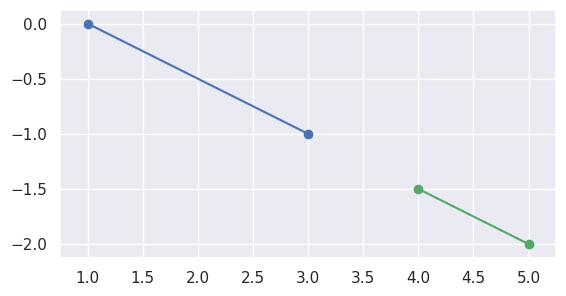>>> start0 = np.asfortranarray([1.0, 0.0])
>>> delta0 = np.asfortranarray([2.0, -1.0])
>>> end0 = start0 + 1.0 * delta0
>>> start1 = start0 + 1.5 * delta0
>>> end1 = start0 + 2.0 * delta0
>>> disjoint, _ = parallel_lines_parameters(start0, end0, start1, end1)
>>> disjoint
True


but if the intervals overlap, like $$\left[0, 1\right]$$ and $$\left[-1, \frac{1}{2}\right]$$, the segments meet: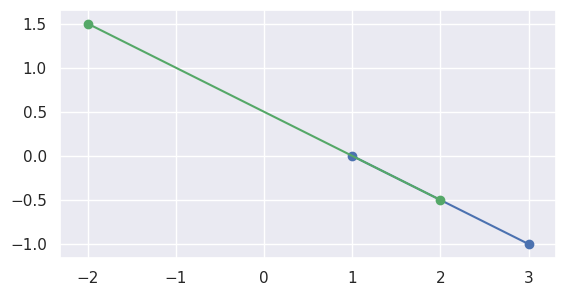>>> start1 = start0 - 1.5 * delta0
>>> end1 = start0 + 0.5 * delta0
>>> disjoint, parameters = parallel_lines_parameters(
...     start0, end0, start1, end1)
>>> disjoint
False
>>> parameters
array([[0.  , 0.5 ],
[0.75, 1.  ]])


Similarly, if the second interval completely contains the first, the segments meet: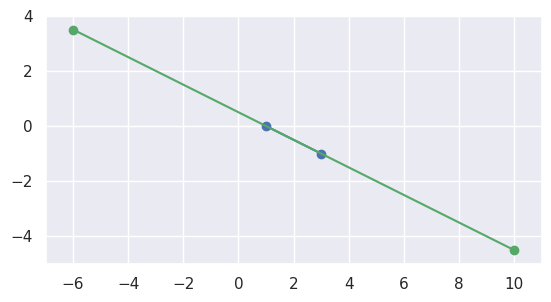>>> start1 = start0 + 4.5 * delta0
>>> end1 = start0 - 3.5 * delta0
>>> disjoint, parameters = parallel_lines_parameters(
...     start0, end0, start1, end1)
>>> disjoint
False
>>> parameters
array([[1.    , 0.    ],
[0.4375, 0.5625]])


Note

This function doesn’t currently allow wiggle room around the desired value, i.e. the two values must be bitwise identical. However, the most “correct” version of this function likely should allow for some round off.

Note

There is also a Fortran implementation of this function, which will be used if it can be built.

Parameters
• start0 (numpy.ndarray) – A 1D NumPy 2-array that is the start vector $$S_0$$ of the parametric line $$L_0(s)$$.

• end0 (numpy.ndarray) – A 1D NumPy 2-array that is the end vector $$E_0$$ of the parametric line $$L_0(s)$$.

• start1 (numpy.ndarray) – A 1D NumPy 2-array that is the start vector $$S_1$$ of the parametric line $$L_1(s)$$.

• end1 (numpy.ndarray) – A 1D NumPy 2-array that is the end vector $$E_1$$ of the parametric line $$L_1(s)$$.

Returns

A pair of

• Flag indicating if the lines are disjoint.

• An optional 2 x 2 matrix of s-t parameters only present if the lines aren’t disjoint. The first column will contain the parameters at the beginning of the shared segment and the second column will correspond to the end of the shared segment.

Return type
bezier.hazmat.geometric_intersection.line_line_collide(line1, line2)

Determine if two line segments meet.

This is a helper for convex_hull_collide() in the special case that the two convex hulls are actually just line segments. (Even in this case, this is only problematic if both segments are on a single line.)

Parameters
• line1 (numpy.ndarray) – 2 x 2 array of start and end nodes.

• line2 (numpy.ndarray) – 2 x 2 array of start and end nodes.

Returns

Indicating if the line segments collide.

Return type

bool

bezier.hazmat.geometric_intersection.convex_hull_collide(nodes1, nodes2)

Determine if the convex hulls of two curves collide.

Note

This is a helper for from_linearized().

Parameters
Returns

Indicating if the convex hulls collide.

Return type

bool

bezier.hazmat.geometric_intersection.from_linearized(first, second, intersections)

Determine curve-curve intersection from pair of linearizations.

Note

This assumes that at least one of first and second is not a line. The line-line case should be handled “early” by check_lines().

Note

This assumes the caller has verified that the bounding boxes for first and second actually intersect.

If there is an intersection along the segments, adds that intersection to intersections. Otherwise, returns without doing anything.

Parameters
• first (Linearization) – First curve being intersected.

• second (Linearization) – Second curve being intersected.

• intersections (list) – A list of existing intersections.

Raises

ValueError – If first and second both have linearization error of 0.0 (i.e. they are both lines). This is because this function expects the caller to have used check_lines() already.

bezier.hazmat.geometric_intersection.add_intersection(s, t, intersections)

Adds an intersection to list of intersections.

Note

This is a helper for from_linearized() and endpoint_check(). These functions are used (directly or indirectly) by all_intersections() exclusively, and that function has a Fortran equivalent.

Accounts for repeated intersection points. If the intersection has already been found, does nothing.

If s is below $$2^{-10}$$, it will be replaced with 1 - s and compared against 1 - s' for all s' already in intersections. (Similar if t is below the ZERO_THRESHOLD.) This is perfectly “appropriate” since evaluating a Bézier curve requires using both s and 1 - s, so both values are equally relevant.

Compares $$\|p - q\|$$ to $$\|p\|$$ where $$p = (s, t)$$ is current candidate intersection (or the “normalized” version, such as $$p = (1 - s, t)$$) and $$q$$ is one of the already added intersections. If the difference is below $$2^{-36}$$ (i.e. NEWTON_ERROR_RATIO) then the intersection is considered to be duplicate.

Parameters
• s (float) – The first parameter in an intersection.

• t (float) – The second parameter in an intersection.

• intersections (list) – List of existing intersections.

bezier.hazmat.geometric_intersection.endpoint_check(first, node_first, s, second, node_second, t, intersections)

Check if curve endpoints are identical.

Note

This is a helper for tangent_bbox_intersection(). These functions are used (directly or indirectly) by all_intersections() exclusively, and that function has a Fortran equivalent.

Parameters
• first (SubdividedCurve) – First curve being intersected (assumed in $$\mathbf{R}^2$$).

• node_first (numpy.ndarray) – 1D 2-array, one of the endpoints of first.

• s (float) – The parameter corresponding to node_first, so expected to be one of 0.0 or 1.0.

• second (SubdividedCurve) – Second curve being intersected (assumed in $$\mathbf{R}^2$$).

• node_second (numpy.ndarray) – 1D 2-array, one of the endpoints of second.

• t (float) – The parameter corresponding to node_second, so expected to be one of 0.0 or 1.0.

• intersections (list) – A list of already encountered intersections. If these curves intersect at their tangency, then those intersections will be added to this list.

bezier.hazmat.geometric_intersection.tangent_bbox_intersection(first, second, intersections)

Check if two curves with tangent bounding boxes intersect.

Note

This is a helper for intersect_one_round(). These functions are used (directly or indirectly) by all_intersections() exclusively, and that function has a Fortran equivalent.

If the bounding boxes are tangent, intersection can only occur along that tangency.

If the curve is not a line, the only way the curve can touch the bounding box is at the endpoints. To see this, consider the component

$x(s) = \sum_j W_j x_j.$

Since $$W_j > 0$$ for $$s \in \left(0, 1\right)$$, if there is some $$k$$ with $$x_k < M = \max x_j$$, then for any interior $$s$$

$x(s) < \sum_j W_j M = M.$

If all $$x_j = M$$, then $$B(s)$$ falls on the line $$x = M$$. (A similar argument holds for the other three component-extrema types.)

Note

This function assumes callers will not pass curves that can be linearized / are linear. In all_intersections(), curves are pre-processed to do any linearization before the subdivision / intersection process begins.

Parameters
• first (SubdividedCurve) – First curve being intersected (assumed in $$\mathbf{R}^2$$).

• second (SubdividedCurve) – Second curve being intersected (assumed in $$\mathbf{R}^2$$).

• intersections (list) – A list of already encountered intersections. If these curves intersect at their tangency, then those intersections will be added to this list.

bezier.hazmat.geometric_intersection.bbox_line_intersect(nodes, line_start, line_end)

Determine intersection of a bounding box and a line.

We do this by first checking if either the start or end node of the segment are contained in the bounding box. If they aren’t, then checks if the line segment intersects any of the four sides of the bounding box.

Note

This function is “half-finished”. It makes no distinction between “tangent” intersections of the box and segment and other types of intersection. However, the distinction is worthwhile, so this function should be “upgraded” at some point.

Parameters
• nodes (numpy.ndarray) – Points (2 x N) that determine a bounding box.

• line_start (numpy.ndarray) – Beginning of a line segment (1D 2-array).

• line_end (numpy.ndarray) – End of a line segment (1D 2-array).

Returns

Enum from BoxIntersectionType indicating the type of bounding box intersection.

Return type

int

bezier.hazmat.geometric_intersection.intersect_one_round(candidates, intersections)

Perform one step of the intersection process.

Note

This is a helper for all_intersections() and that function has a Fortran equivalent.

Checks if the bounding boxes of each pair in candidates intersect. If the bounding boxes do not intersect, the pair is discarded. Otherwise, the pair is “accepted”. Then we attempt to linearize each curve in an “accepted” pair and track the overall linearization error for every curve encountered.

Parameters
Returns

Returns a list of the next round of candidates.

Return type

list

bezier.hazmat.geometric_intersection.prune_candidates(candidates)

Reduce number of candidate intersection pairs.

Note

This is a helper for all_intersections().

Uses more strict bounding box intersection predicate by forming the actual convex hull of each candidate curve segment and then checking if those convex hulls collide.

Parameters

candidates (List [ Union [ SubdividedCurve, Linearization ] ]) – An iterable of pairs of curves (or linearized curves).

Returns

A pruned list of curve pairs.

Return type
bezier.hazmat.geometric_intersection.make_same_degree(nodes1, nodes2)

Degree-elevate a curve so two curves have matching degree.

Parameters
• nodes1 (numpy.ndarray) – Set of control points for a Bézier curve.

• nodes2 (numpy.ndarray) – Set of control points for a Bézier curve.

Returns

The potentially degree-elevated nodes passed in.

Return type
bezier.hazmat.geometric_intersection.coincident_parameters(nodes1, nodes2)

Check if two Bézier curves are coincident.

Does so by projecting each segment endpoint onto the other curve

$\begin{split}B_1(s_0) = B_2(0) \\ B_1(s_m) = B_2(1) \\ B_1(0) = B_2(t_0) \\ B_1(1) = B_2(t_n)\end{split}$

and then finding the “shared interval” where both curves are defined. If such an interval can’t be found (e.g. if one of the endpoints can’t be located on the other curve), returns None.

If such a “shared interval” does exist, then this will specialize each curve onto that shared interval and check if the new control points agree.

Parameters
• nodes1 (numpy.ndarray) – Set of control points for a Bézier curve.

• nodes2 (numpy.ndarray) – Set of control points for a Bézier curve.

Returns

A 2 x 2 array of parameters where the two coincident curves meet. If they are not coincident, returns None.

Return type
bezier.hazmat.geometric_intersection.check_lines(first, second)

Checks if two curves are lines and tries to intersect them.

Note

This is a helper for all_intersections().

If they are not lines / not linearized, immediately returns False with no “return value”.

If they are lines, attempts to intersect them (even if they are parallel and share a coincident segment).

Parameters
Returns

A pair of

• Flag indicating if both candidates in the pair are lines.

• Optional “result” populated only if both candidates are lines. When this result is populated, it will be a pair of

• array of parameters of intersection

• flag indicating if the two candidates share a coincident segment

Return type
bezier.hazmat.geometric_intersection.all_intersections(nodes_first, nodes_second)

Find the points of intersection among a pair of curves.

Note

There is also a Fortran implementation of this function, which will be used if it can be built.

Note

This assumes both curves are in $$\mathbf{R}^2$$, but does not explicitly check this. However, functions used here will fail if that assumption fails, e.g. bbox_intersect() and newton_refine().

Parameters
• nodes_first (numpy.ndarray) – Control points of a curve to be intersected with nodes_second.

• nodes_second (numpy.ndarray) – Control points of a curve to be intersected with nodes_first.

Returns

An array and a flag:

• A 2 x N array of intersection parameters. Each row contains a pair of values $$s$$ and $$t$$ (each in $$\left[0, 1\right]$$) such that the curves intersect: $$B_1(s) = B_2(t)$$.

• Flag indicating if the curves are coincident.

Return type
Raises
• ValueError – If the subdivision iteration does not terminate before exhausting the maximum number of subdivisions.

• NotImplementedError – If the subdivision process picks up too many candidate pairs. This typically indicates tangent curves or coincident curves (though there are mitigations for those cases in place).

class bezier.hazmat.geometric_intersection.BoxIntersectionType

Bases: object

Enum representing all possible bounding box intersections.

Note

This class would be more “correct” as an enum.Enum, but it we keep the values integers to make interfacing with Fortran easier.

INTERSECTION = 0

Bounding boxes overlap with positive area.

TANGENT = 1

Bounding boxes are tangent but do not overlap.

DISJOINT = 2

Bounding boxes do not intersect or touch.

class bezier.hazmat.geometric_intersection.SubdividedCurve(nodes, original_nodes, start=0.0, end=1.0)

Bases: object

A data wrapper for a Bézier curve

To be used for intersection algorithm via repeated subdivision, where the start and end parameters must be tracked.

Parameters
nodes
original_nodes
start
end
subdivide()

Split the curve into a left and right half.

See Curve.subdivide() for more information.

Returns

The left and right sub-curves.

Return type
class bezier.hazmat.geometric_intersection.Linearization(curve, error)

Bases: object

A linearization of a curve.

This class is provided as a stand-in for a curve, so it provides a similar interface.

Parameters
curve

The curve that this linearization approximates.

Type

SubdividedCurve

error

The linearization error for the linearized curve.

Type

float

start_node

The 1D start vector of this linearization.

Type

numpy.ndarray

end_node

The 1D end vector of this linearization.

Type

numpy.ndarray

subdivide()

Do-nothing method to match the Curve interface.

Returns

List of all subdivided parts, which is just the current object.

Return type
classmethod from_shape(shape)

Try to linearize a curve (or an already linearized curve).

Parameters

shape (Union [ SubdividedCurve, Linearization ]) – A curve or an already linearized curve.

Returns

The (potentially linearized) curve.

Return type